## Pages

### What is the purpose of Hall Effect?

It is often necessary to determine whether a material is P-type or n-type. Simply by measuring conductivity we cannot distinguish between hole and electron conduction.
The Hall Effect can be utilized to distinguish between two types of carriers, and it also allows the density of charge carriers to be determined.

### Principle

If a piece of semi conductor carrying a current is placed in a transverse magnetic field, an electric field is produced inside the conductor in a direction normal to both current and magnetic field. This effect is known as Hall Effect and generated voltage is called Hall Voltage.

Consider an n-type semiconductor slab; the current flow consists off almost entirely of electrons moving from right to left. This corresponds to direction of conventional current form left to right.

If ‛V’ is velocity of electrons at right angle to magnetic field, there is a down ward force on each electron of magnitude Bev. This causes the electron current to be deflected in a downward direction and causes a negative charge to accumulate on bottom face of slab. A Potential Difference is established from top to bottom of specimen with bottom face negative. This Potential Difference causes Electric field ‛EH’ in negative ‘Y’ direction and therefore a force ‘eEH’acting in upward direction on electron.
Equilibrium occurs when
eEH = BeV     ------------------- (1)
EH  = Bv   -------------------------(2)
If  Jx is current density in X – direction then
Jx= neV;

Where
‘V’ is average drift velocity,
‘n’ is concentration of current carriers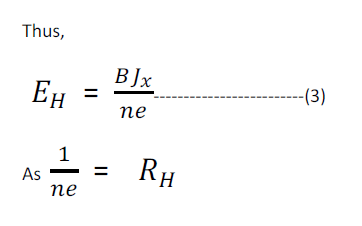The hall effect is described by means off Hall coeff 'RH', defined in terms of current density 'Jx' by relation.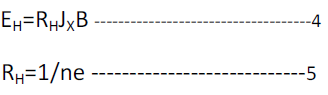In this case RH=1/ne; negative sign is used because electric field developed is in negative Y-direction.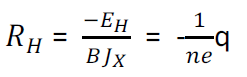All 3 quantities EH, B and Jcan be measured and so the hall coeff and carrier density ‛n’ can be found out.

Determination of Hall Coefficient

The Hall Coefficient is determined by measuring the Hall voltage that generates the Hall field. If ‛VH’ is Hall voltage across sample of thickness ‛t’, then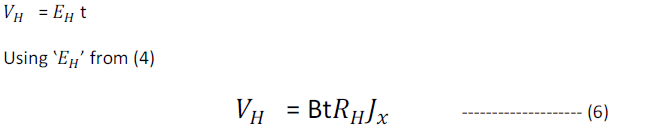If ‛b’ is width of sample then is cross section will be ‛bt’ and the current density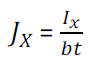Thus,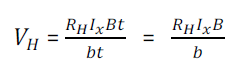Note: - the polarity off VH’ will be opposite for ‛n’ type and ‛P’ type semiconductors.

There is another interesting quantity called Hall angle defined by eqn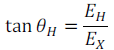### Applications of Hall Effect

Determination of semiconductor Type
For a n-type semiconductor, the Hall coefficient is negative where as for a p-type semiconductor it is positive. Thus, the sign of Hall coeff can be used to determine whether a given semi conductor is ‘n’ or ‘p’ type.

Calculation of carrier concentration

The hall voltage 'VH' is measured as usual by placing the two probes at centers of top & bottom faces of sample.

Measurement of magnetic flux density

Since Hall voltage is proportional to magnetic flux density ‛B’ for a given current Ix thru a sample, the Hall effect can be used as basis for the design of a magnetic flux density meter.

Hall Effect multiplier
If the magnetic flux density ‛B’ is produced by passing a current I’ thru an air core coil, ‛B’ will be proportional to I’. The Hall voltage is thus proportional to product of I & I’. This forms the basis of multiplier.

#### Measurement of Hall Voltage helps in the following

1. The sign of current carrying charges can be determined.
2. The number of charge carriers present in unit volume can be calculated from magnitude of RH.
3. Mobility of charge carriers may be obtained directly from Hall Voltage.